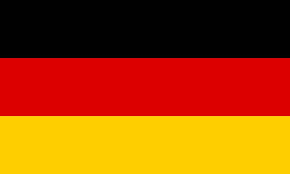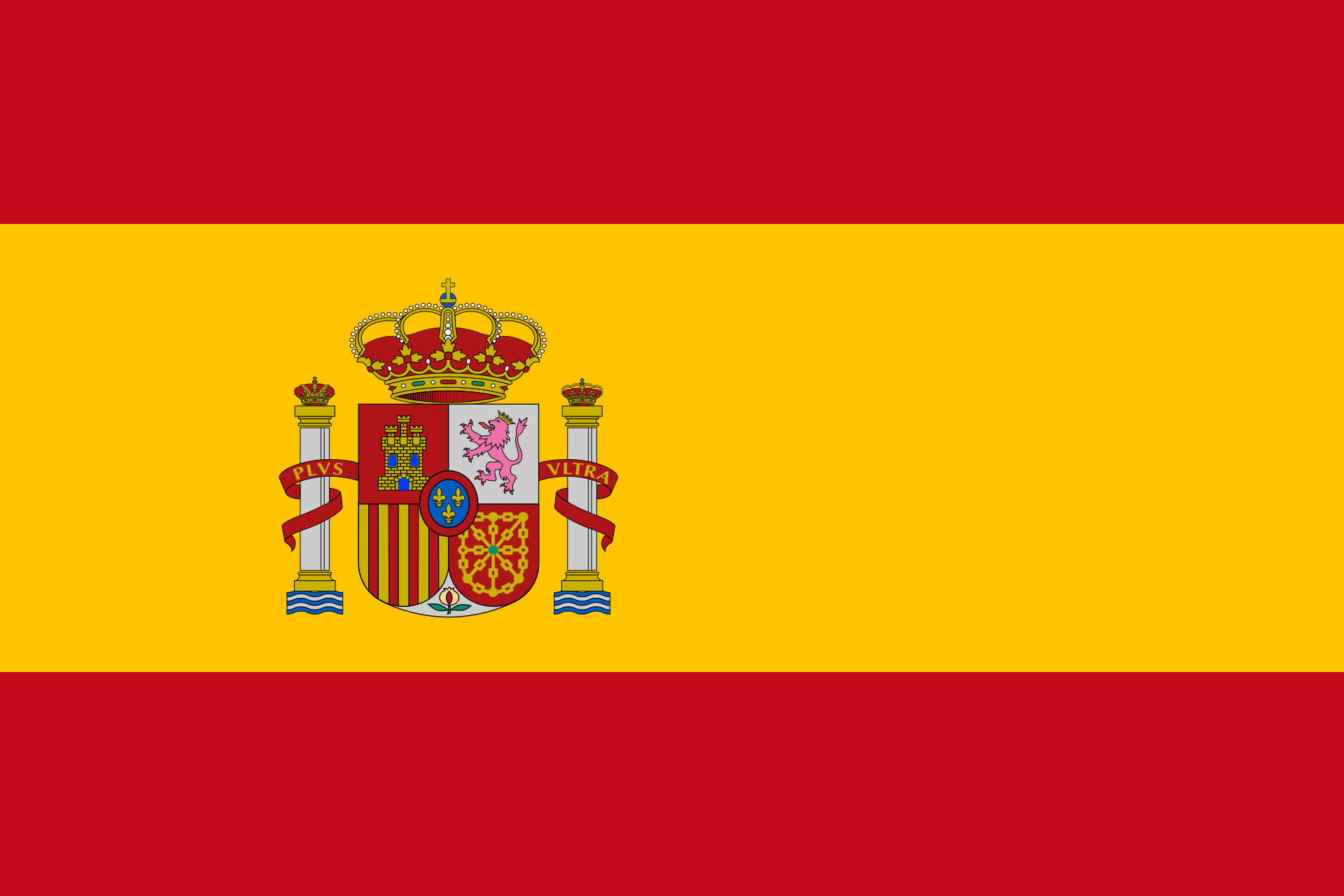# Line intersectionChoose how the first line is given.
• parametric equation:

 g: x =+ r• Given through two points

to be equalized with line

Choose how the second line is given.
• parametric equation:

 g: x =+ r• Given through two points

## What's this about?

In the plane, lines can just be parallel, intersecting or equal. In space, there is another possibility: Lines can be not parallel but also not intersecting because one line is going over the other one in some way. This is called skew.

## How to find how lines intersect?

The best way is to check the directions of the lines first. If they are the same, the lines can just be parallel or identical. If not, you check for an intersection point. If no such point exists, the lines have to be skew.

## How to find out what is the case for my lines?

Just enter the lines above. Mathepower does the calculation immediately, for free and step-by-step. No need to register or something.

## How to illustrate lines in vector geometry?

For a line, you need a point and a direction. The point is just any point on the line (therefore you got infinitely many possibilities which vector to take.) The direction is from one point of the line to any other point. The line has infinitely many points, so you got infinitely many possibilities which direction vector to take. All directions of a line are collinear.